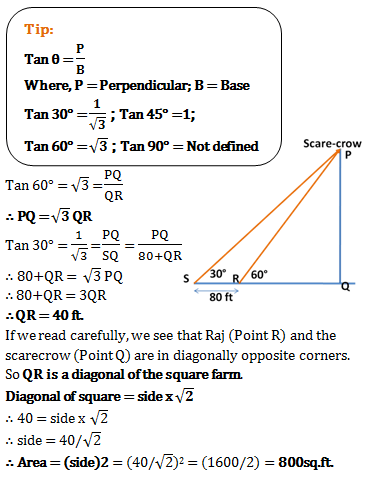# Mensuration and Trigonometry - Aptitude Questions and Answers - Set 2

1)   A man walks entirely around his semi-circular garden. What is the area of his garden, if he walks 324m?

a. 18 sq. m.
b. 6237 sq.m.
c. 5248 sq. m.
d. 5368 sq. m.
 Answer  Explanation ANSWER: 6237 sq.m. Explanation: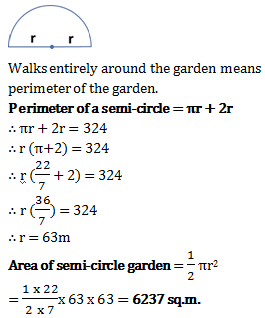2)   Ramesh runs along a circular park every day at a speed of 60m/min. One day he runs along the diameter with same speed and crosses the park. He needed 60 minutes less to cross the park. What is the radius of the park?

a. 1680 m
b. 3600 m
c. 1750 m
d. 1530 m
 Answer  Explanation ANSWER: 1680 m Explanation: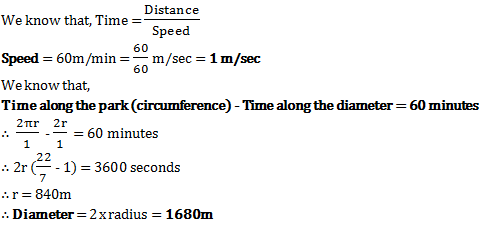3)   A semicircle of 8 cm diameter has 3 equilateral triangles inscribed within it. What is the total area of the triangles?

a. 72 sq.cm.
b. 192 sq.cm.
c. 432 sq.cm.
d. 122 sq.cm.
 Answer  Explanation ANSWER: 432 sq.cm. Explanation: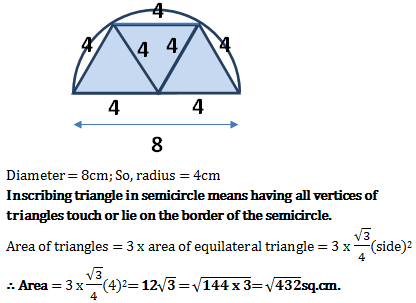4)   Length of a rectangle is 12cm more than its breadth and it has a perimeter of 200cm. What will be diameter of a circle whose area matches the area of this rectangle?

a. 44 cm
b. 56 cm
c. 28 cm
d. 45 cm
 Answer  Explanation ANSWER: 56 cm Explanation: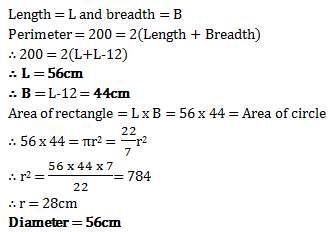5)   If a circle and a square have the same area, then what will be the perimeter of the square, if diameter of the circle is 8 cm.

a.
b. 32
c. 4π
d. 16π
 Answer  Explanation ANSWER: 16π Explanation: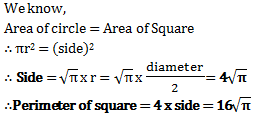6)   A field with equal sides of 40m is full of grass. One cow is tied to each corner of the field by a rope of 14m. How much grass will not be eaten by those 4 cows?

a. 984 sq.m
b. 1056 sq.m
c. 1224 sq.m
d. 856 sq.m
 Answer  Explanation ANSWER: 984 sq.m Explanation: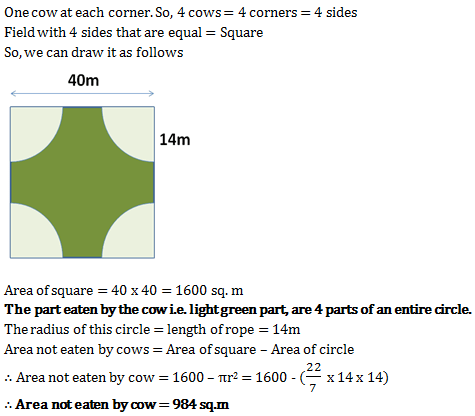7)   Baskin n Robbin’s biggest ice-cream cardboard cone has height of 24 cm and slant height of 25cm. One sheet of cardboard makes 8 such cones. What is area of the sheet?

a. 4400 sq. cm
b. 6236 sq. cm
c. 6000 sq. cm
d. 550 sq. cm
 Answer  Explanation ANSWER: 4400 sq. cm Explanation: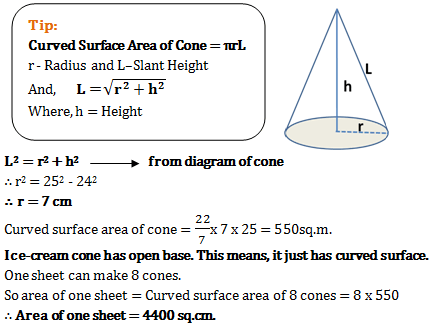8)   Suresh wanted to keep volume of his right circular cone shaped cap same, but increase its height to 9 times. By how much should he increase or decrease the radius?

a. Increase to 3 times
b. Reduce to 1/3rd
c. Increase by 18 times
d. Reduce to 1/9th
 Answer  Explanation ANSWER: Reduce to 1/3rd Explanation: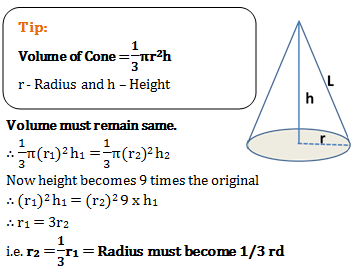9)   A sphere with surface area (792/7) sq.m. has a volume ____

a. 7 sq.cm.
b. 792 cu.cm.
c. 792/7 cu.cm.
d. 792π/7 cu.cm.
 Answer  Explanation ANSWER: 792/7 cu.cm. Explanation: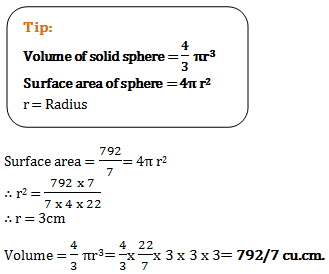10)   A solid rubber sphere weighs 6kg when its diameter is 6cm.  Using the same material, a hollow sphere is made with outer diameter 18cm and inner diameter 12 cm. What is its weight?

a. 114 kg
b. 96 kg
c. 72.64 kg
d. 64 33 kg
 Answer  Explanation ANSWER: 114 kg Explanation: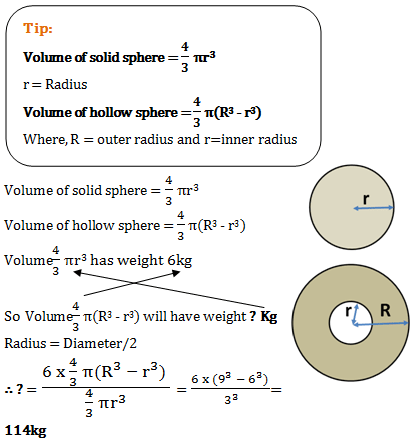11)   2 cm thick 14 metal plates are kept exactly one above the other. On the top plate a hemisphere of diameter 6cm is kept. This just covers the top plate. What is the volume of the entire object?

a. 360π cu.cm.
b. 144π cu.cm.
c. 81 cu.cm
d. 270π cu.cm.
 Answer  Explanation ANSWER: 270π cu.cm. Explanation: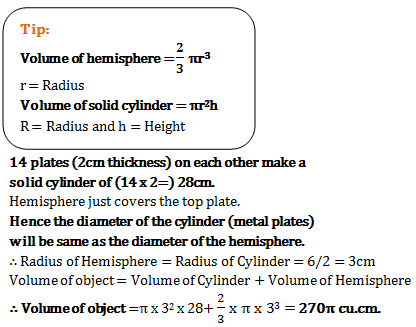12)   Ramesh’s bedroom has a rectangular floor. He built the 4 walls of this room in Rs. 24000 at the rate of Rs. 20 per sq. m. What is height of his bedroom if perimeter of the floor is 150m?

a. 5 m
b. 8 m
c. 15 m
d. 16 m
 Answer  Explanation ANSWER: 8 m Explanation: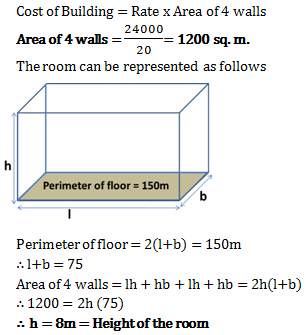13)   Rohit while seeing a bird on tree top made 45° angle of elevation. He walks 240ft. towards the tree to observe the bird closely, thus making 60° angle of elevation. How far was Rohit from the tree initially?

a. (2403)/(3-1) ft
b. 240/(3-1) ft
c. 240/3 ft
d. 2403 ft
 Answer  Explanation ANSWER: (2403)/(3-1) ft Explanation: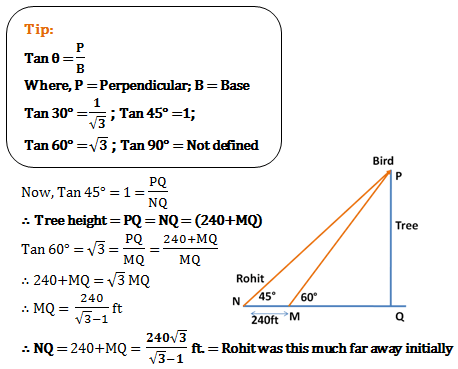14)   Ramesh and Suresh’s mud forts have heights 8cm and 15 cm. They are 24 cm apart. How far are the fort tops from each other?

a. 31 cm
b. 24 cm
c. 25 cm
d. 24.5 cm
 Answer  Explanation ANSWER: 25 cm Explanation: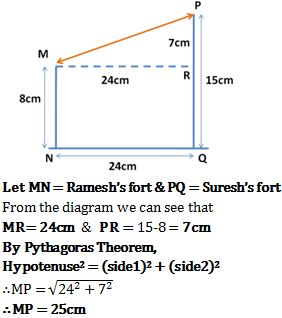15)   Angles of elevation of pole are 60° and 45° from points at distances m and n on ground respectively. Here m, when measured from base of pole is less than n. What is the height of the pole?

a.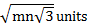b.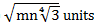c. 3mn units
d. mn units
 Answer  Explanation ANSWER:Explanation: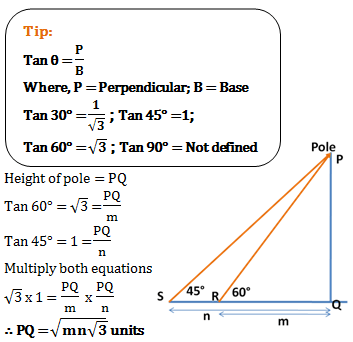16)   Raj stands in a corner of his square farm. Angle of elevation of a scarecrow placed in diagonally opposite corner is 60°. He starts walking backwards in a straight line and after 80ft he realizes that angle of elevation of the scarecrow now is 30°. What is area of the field?

a. 1600 sq.ft.
b. 40 sq.ft.
c. 40/2 sq.ft.
d. 800 sq.ft.
 Answer  Explanation ANSWER: 800 sq.ft. Explanation: